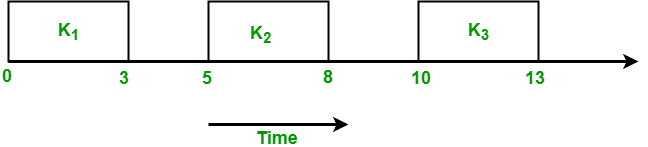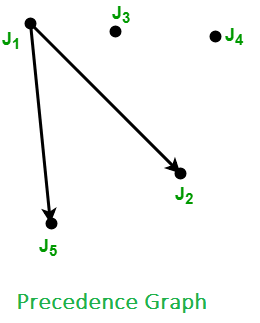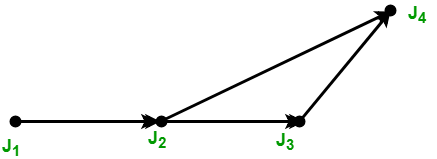# Tasks in Real Time systems

The system is subjected to real time, i.e. response should be guaranteed within a specified timing constraint or system should meet the specified deadline. For example flight control system, real-time monitors etc.
There are two types of tasks in real-time systems:

• Periodic Tasks: In periodic task, jobs are released at regular intervals. A periodic task is one which repeats itself after a fixed time interval. A periodic task is denoted by five tuples: Ti = < Φi, Pi, ei, Di >
Where,

• Φi – is the phase of the task. Phase is release time of the first job in the task. If the phase is not mentioned then release time of first job is assumed to be zero.
• Pi – is the period of the task i.e. the time interval between the release times of two consecutive jobs.
• ei – is the execution time of the task.

For example: Consider the task Ti with period = 5 and execution time = 3
Phase is not given so, assume the release time of the first job as zero. So the job of this task is first released at t = 0 then it executes for 3s and then next job is released at t = 5 which executes for 3s and then next job is released at t = 10. So jobs are released at t = 5k where k = 0, 1, . . ., nHyper period of a set of periodic tasks is the least common multiple of periods of all the tasks in that set. For example, two tasks T1 and T2 having period 4 and 5 respectively will have a hyper period, H = lcm(p1, p2) = lcm(4, 5) = 20. The hyper period is the time after which pattern of job release times starts to repeat.

• Dynamic Tasks: It is a sequential program that is invoked by the occurrence of an event. An event may be generated by the processes external to the system or by processes internal to the system. Dynamically arriving tasks can be categorized on their criticality and knowledge about their occurrence times.
Where
ei – the execution time of the task.
gi – the minimum separation between the occurrence of two consecutive instances of the task.

Jitter: Sometimes actual release time of a job is not known. Only know that ri is in a range [ ri-, ri+ ]. This range is known as release time jitter. Here ri– is how early a job can be released and ri+ is how late a job can be released. Only range [ ei-, ei+ ] of the execution time of a job is known. Here ei– is the minimum amount of time required by a job to complete its execution and ei+ the maximum amount of time required by a job to complete its execution.

Precedence Constraint of Jobs: Jobs in a task are independent if they can be executed in any order. If there is a specific order in which jobs in a task have to be executed then jobs are said to have precedence constraints. For representing precedence constraints of jobs a partial order relation < is used. This is called precedence relation. A job Ji is a predecessor of job Jj if Ji < Jj i.e. Jj cannot begin its execution until Ji completes. Ji is an immediate predecessor of Jj if Ji < Jj and there is no other job Jk such that Ji < Jk < Jj. Ji and Jj are independent if neither Ji < Jj nor Jj < Ji is true.
An efficient way to represent precedence constraints is by using a directed graph G = (J, <) where J is the set of jobs. This graph is known as the precedence graph. Jobs are represented by vertices of graph and precedence constraints are represented using directed edges. If there is a directed edge from Ji to Jj then it means that Ji is immediate predecessor of Jj. For example: Consider a task T having 5 jobs J1, J2, J3, J4 and J5 such that J2 and J5 cannot begin their execution until J1 completes and there are no other constraints.
The precedence constraints for this example are:
J1 < J2 and J1 < J5Set representation of precedence graph:

1. < (1) = { }
2. < (2) = {1}
3. < (3) = { }
4. < (4) = { }
5. < (5) = {1}

Consider another example where precedence graph is given and you have to find precedence constraintsFrom the above graph, we derive the following precedence constraints:

1. J1 < J2
2. J2 < J3
3. J2 < J4
4. J3 < J4

Attention reader! Don’t stop learning now. Get hold of all the important CS Theory concepts for SDE interviews with the CS Theory Course at a student-friendly price and become industry ready.

My Personal Notes arrow_drop_upCheck out this Author's contributed articles.

If you like GeeksforGeeks and would like to contribute, you can also write an article using contribute.geeksforgeeks.org or mail your article to contribute@geeksforgeeks.org. See your article appearing on the GeeksforGeeks main page and help other Geeks.

Please Improve this article if you find anything incorrect by clicking on the "Improve Article" button below.

Improved By : dcbleo

Article Tags :
Practice Tags :

9

Please write to us at contribute@geeksforgeeks.org to report any issue with the above content.